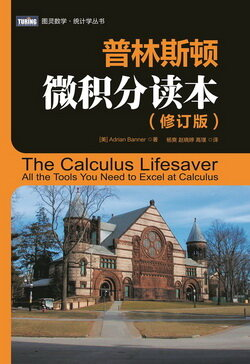# 普林斯顿微积分读本（修订版）(20)：三角学回顾 2.4(三角恒等式)

$\tan(x)=\frac{\sin(x)}{\cos(x)},\quad\cot(x)=\frac{\cos(x)}{\sin(x)}.$

(有时, 根据这些恒等式, 用正弦和余弦来代替每一个正切和余切会有帮助, 但这只是你被卡住时不得已而为之的下下策.)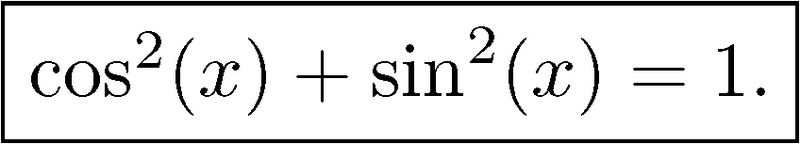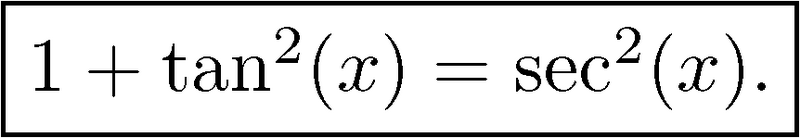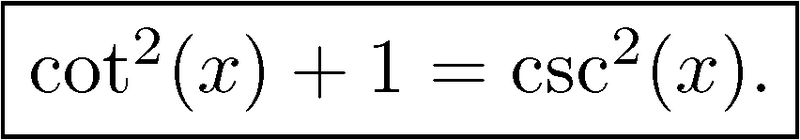$\sin(x)=\cos\Bigl(\frac{\pi}{2}-x\Bigr),\quad\tan(x)=\cot\Bigl(\frac{\pi}{2}-x\Bigr),\quad\sec(x)=\csc\Bigl(\frac{\pi}{2}-x\Bigr).$

$\cos(x)=\sin\Bigl(\frac{\pi}{2}-x\Bigr),\quad\cot(x)=\tan\Bigl(\frac{\pi}{2}-x\Bigr),\quad\csc(x)=\sec\Bigl(\frac{\pi}{2}-x\Bigr).$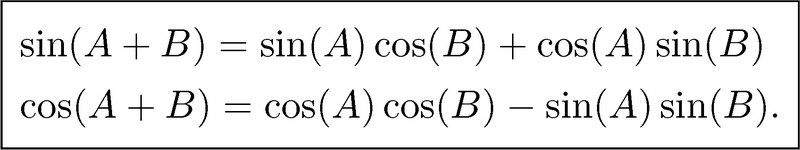\begin{aligned}&\sin(A+B)=\sin(A)\cos(B)+\cos(A)\sin(B)\&\cos(A+B)=\cos(A)\cos(B)-\sin(A)\sin(B).\end{aligned}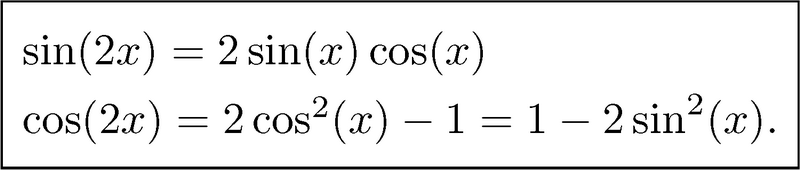$\sin(4x)=2(2\sin(x)\cos(x))(2\cos^2(x)-1)=8\sin(x)\cos^3(x)-4\sin(x)\cos(x).$

$\cos(4x)=2\cos^2(2x)-1=2(2\cos^2(x)-1)^2-1=8\cos^4(x)-8\cos^2(x)+1.$